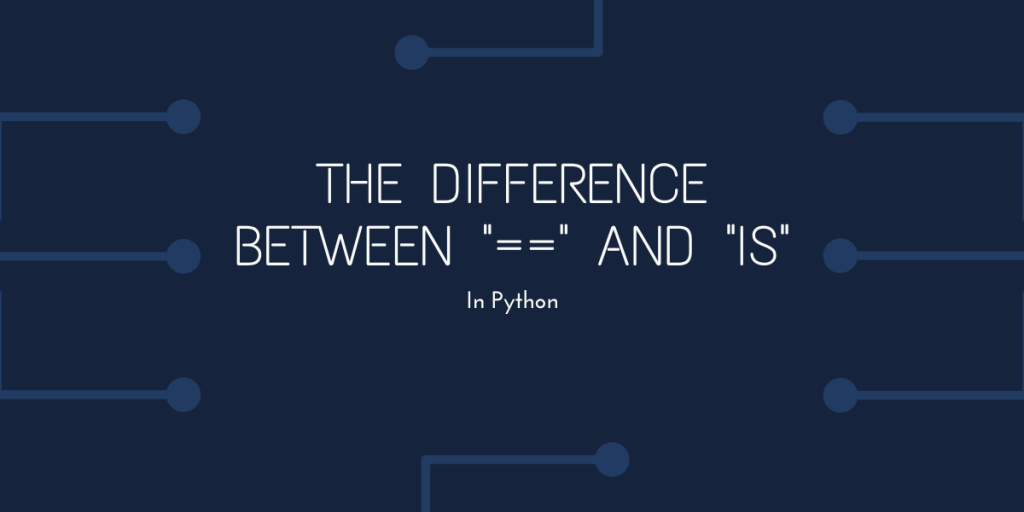# The difference between == and is in PythonThe operators `==` and `is` both perform very similar tasks in Python, but they are very different from each other and deal with a very interesting concept: how Python stores its variables in memory.

## Understanding the Difference between == and is

In simple terms,

• `==` checks the value of the two operands, and if they’re the same, it returns `True`, otherwise, it returns `False`.
• `is` checks the object ID of the two operands, and returns `True` if they’re the same.

But what is object ID? Every object is allocated an ID in memory, and two variables can point to the same object, which gives them the same object ID.

Let us use lists to see their difference:

```lst1 = [1,2,3]
lst2 = [1,2,3]
print(lst1 == lst2)
print(lst1 is lst2)

lst2 = lst1
print()
print(lst1 is lst2)
```

Output:

``````True
False

True``````

In the above case, initially, there are two lists in memory, but they hold the exact same values.

• `==` compares values, so that operation returns `True`.
• But `is` checks if they point to the same object, and these lists are actually different objects, so that returns `False`.
• After this, we make `lst2` equal to `lst1`, this makes `lst2` point to where `lst1` is pointing, so then, `is` returns `True`.

All of this is very different for integers, and to understand that, we need to know how objects are stored in memory.

## Memory Allocation in Python

We need to understand the memory allocation of objects in Python in order to go further in this tutorial.

Let us take an example:

```number = 10
```

Here, we know that `number` holds the value 10, but how is it stored in memory? In Python, every object has four parts in the memory:

1. Size – For every object, 4 bytes are reserved that hold the size of the object.
2. Reference Count – For every object, 8 bytes are reserved that hold the number of variables that are pointing to this object. And all these objects will have the object ID of this object.
3. Object Type – For every object, 8 bytes are reserved that hold the information that says which type of object it is.
4. Object Value – Another 8 bytes are reserved for every object and they hold the object’s actual value.

Now, in the above list, object value and reference count are the ones that are important for this discussion.

So, for `number = 10`, there is an object in the memory that has object value 10 and reference count 1, which means the object has a value of 10 and one variable is pointing towards it, which in our case is `number`.

Now let’s say we declare another variable like this:

```number_2 = number
print(number == number_2)
print(number is number_2)
```

Now, a very interesting thing will happen in the memory, there will be no new objects created, and `number_2` will also point to where `number` is pointing, and the reference count of the object will increase to 2.

Consequently, `number` and `number_2` will have the same object ID, and the output will be:

``````True
True``````

But what if we do this?

```num1 = 10
num2 = 10
print(num1 == num2)
print(num1 is num2)
```

In the above example, the output will be:

``````True
True``````

## The special numbers from -5 to 256

Python considers the integers from -5 to 256 commonly used integers, and so the objects for these integers are always predefined and you cannot remove those objects from memory.

So at the beginning of a Python code, all these objects have a reference count of zero, but if any variables are made to hold values between -5 and 256 (inclusive), then no new objects will be created and all the variables will just point to the already existing ones.

So in the above code, the object for 10 is already there in the memory, the first two lines just create variables that point to that same object.

So, for the following code:

```num1 = 257
num2 = 257
print(num1 == num2)
print(num1 is num2)
```

The output will be:

``````True
False``````

This is because python creates new instances for integers outside that range every time they are declared. Note that if we would have done `num2 = num1`, then no matter the value of `num1`, both of them will point to the same object.

## Conclusion

In this tutorial, we saw the difference between `==` and `is`, and how `is` can be useful in finding if two variables point to the same memory location.

We also saw how the integers from -5 to 256 are cached in the memory because they are considered commonly used (characters like `'a'` and `'b'` are also cached similarly), and two variables having the same value in this range will be pointing to the same object.